GeeksforGeeks App
Open AppBrowser
Continue

# How to Remove Noise from Digital Image in Frequency Domain Using MATLAB?

Noise is defined as aberrant pixels. In other words, noise is made up of pixels not correctly representing the color or exposure of the scene. In this article, we will how to remove noise from the digital images in the frequency domain.

There are two types of Noise sources

• Image acquisition
• Image transmission

In digital image processing different types of Noise models are available namely:

• Spatially independent noise models
• Gaussian noise
• Rayleigh noise
• Erlang (Gamma) noise
• Exponential noise
• Impulse (salt-and-pepper) noise
• A spatially dependent noise model
• Periodic noise

Now we see the removal of Gaussian noise from images.

Steps:

• Create the white Gaussian noise and add it to the image.
• Compute the Fourier Transformation of the noisy image.
• Create the Butterworth Low Pass Filter.
• Take the element-wise multiplication of BLPF and FT of a noisy image.
• Compute the inverse FT of the above result.
• Display the denoised image along with intermediate images.

Function Used:

• imtool( ) inbuilt function is used to display the image.
• rgb2gray( ) inbuilt function is used to convert RGB image into grayscale image.
• randn( ) inbuilt function is used to create random noise of specified standard deviation.
• double( ) inbuilt function is used to convert integer value into the double format.
• fft2( ) inbuilt function is used to perform 2-D Fourier Transformation.
• fftshift( ) inbuilt function is used to move corners to the center.
• meshgrid( ) inbuilt function is used to create the 2-D grid containing the coordinates of the vectors.
• ifft2( ) inbuilt function is used to perform inverse Fourier transformation.
• sqrt( ) inbuilt function is used to calculate the square root of the given inputs.

Example:

## Matlab

 `%MATLAB CODE For``% REMOVAL OF GAUSSIAN``% NOISE IN FREQUENCY DOMAIN.` `function` `RemoveGaussianNoise(img)` `%convert into grayscale if not.``[M,N,D]=size(img)``if``(D==3)``    ``k=rgb2gray(img);``end` `%display image.``imtool(k, []);` `%define noise.``n=15*randn(size(k));``kn=double(k)+n;` `%display noised image.``imtool(kn,[]);` `%Convert into Frequency domain.``ft=fft2(kn);` `%display centered FT spectrum.``imtool(abs(log(fftshift(ft))),[]);` `% MATLAB Code | Butterworth Low Pass Filter    ``% Assign the order value``n = 2; ``% one can change this value accordingly ` `% Assign Cut-off Frequency``D0 = 20; ``% one can change this value accordingly``  ` `% Designing filter``u = 0:(M-1);``v = 0:(N-1);``idx = find(u > M/2);``u(idx) = u(idx) - M;``idy = find(v > N/2);``v(idy) = v(idy) - N;``  ` `% MATLAB library function meshgrid(v, u) returns``% 2D grid which contains the coordinates of vectors``% v and u. Matrix V with each row is a copy of v``% and matrix U with each column is a copy of u``[V, U] = meshgrid(v, u);``  ` `% Calculating Euclidean Distance``D = sqrt(U.^2 + V.^2);``  ` `% determining the filtering mask``H = 1./(1 + (D./D0).^(2*n));` `%Display BLPF.``imtool(fftshift(H),[]);` `%Perform element wise multiplication.``res=H.*ft;` `%take inverse FT.``dn=ifft2(res);` `%Display denoised image.``imtool(dn,[]);``end` `%%%%%UTILITY CODE%%%%``img=imread(``"cameraman.png"``);``RemoveGaussianNoise(img);`

Output: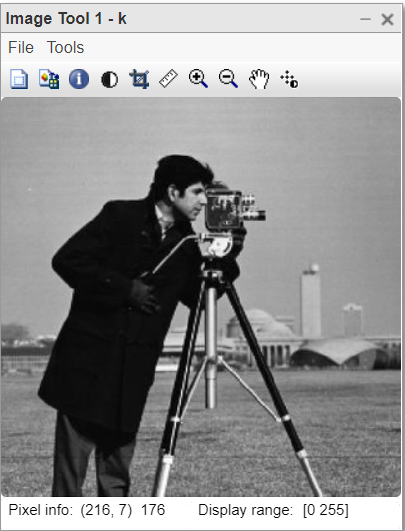Figure 1: Original input image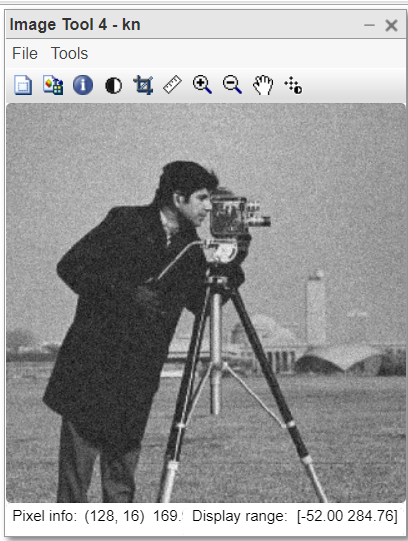Figure 2: Noisy image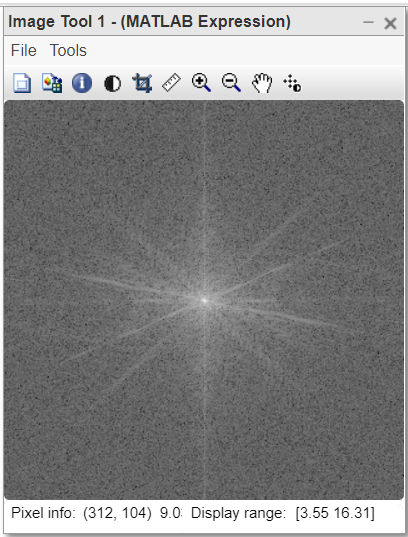Figure 3: Fourier Transform centred spectrum of the noisy image.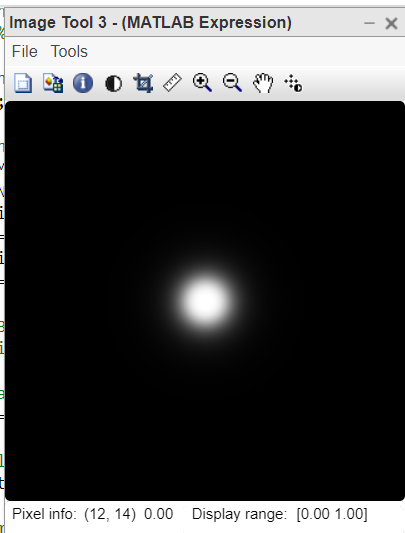Figure 5: Denoised Image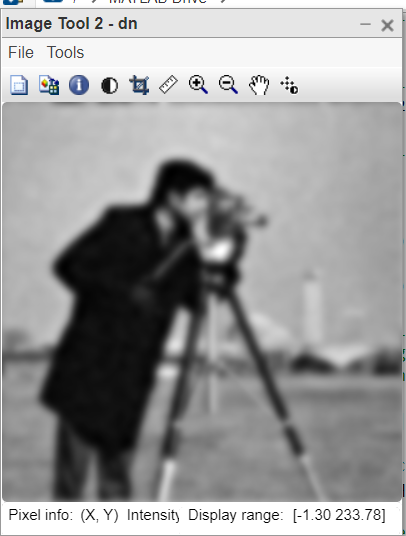Figure 6: Denoised Image

The Gaussian noise is best removed in the spatial domain using the Gaussian filter and NLM filter. We can try to reduce the Gaussian noise in the frequency domain using Butterworth LPF but the result is not very effective. It does reduce the noise to some extent but at the cost of blurriness. The resultant image is noise-free but blurriness occurs in the image which makes it difficult to observe finer details in the image.

Now we see the removal of periodic noise from Image in the frequency domain. So the noise which is present in the repetitive pattern is called periodic noise. It is seen as lines in the image. The source of periodic noise is electrical or electromechanical interference during capturing the image. Periodic noise after converting into the frequency domain is seen as discrete spikes in the image. To remove this type of noise, we have to use notch filters in the frequency domain. After applying notch filters, some noise still remains at the corners.

Steps:

• Compute the Fourier Transform of the image.
• Get the centered FT spectrum and display.
• Spot the periodic noise pattern in the FT image.
• Block the periodic noise pattern in the FT image.
• Convert the image back into the spatial domain from the frequency domain.
• Compute the inverse Fourier transform of the image.
• Display the image.

Syntax:

• imtool( ) inbuilt function is used to display the image.
• fft2( ) inbuilt function is used to perform fourier transform of image in 2D.
• fftshift( ) inbuilt function is used to get centered FT spectrum.
• ifft2( ) inbuilt function is used to perform inverse Fourier transform.
• ifftshift( ) inbuilt function is used to decentered the FT spectrum.
• abs( ) inbuilt function is used to absolute value.
• log( ) inbuilt function is used to get logarithm of the value.

Example:

## Matlab

 `% MATLAB CODE``%REMOVAL OF PERIODIC NOISE FROM IMAGE.` `function` `RemovePeriodicNoise(img)``% take FT of image and``% shift corners to center.``Fourier_transform=fft2(img);``Centered_shifted=fftshift(Fourier_transform);` `% Block the noise spectrum.``Centered_shifted(1:125,110:130)=0;``%for man``Centered_shifted(190:320,110:130)=0;``%diagonals` `Centered_shifted(120:x,1:100)=0;``%for lady with hat.``Centered_shifted(1:100,120:y)=0;``%diagonals.` `% inverse shift center to``% corner and take Inverse FT.``Inverse_shifted=ifftshift(Centered_shifted);``Output_image=ifft2(Inverse_shifted);` `% display input image.``original_input_image=img;``imtool(original_input_image,[]);` `% display FT spectrum.``Centered_shifted_spectrum=abs(log(fftshift(Fourier_transform)));``imtool(Centered_shifted_spectrum,[]);` `% display denoised FT.``Noise_free_FT=log(Centered_shifted);``imtool(Noise_free_FT,[]);` `% display output image.``imtool(abs(Output_image),[]);``end` `%%%UTILITY CODE%%%``k=imread(``"periodic_noise1.png"``);``RemovePeriodicNoise(k);`

Output: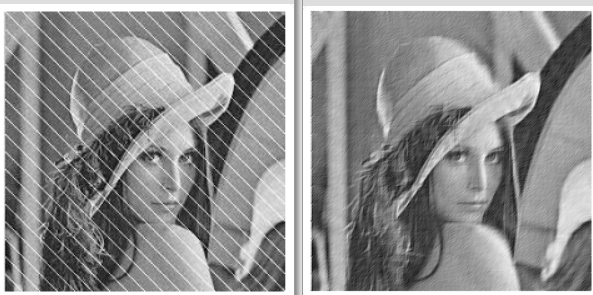Figure 7: Input and Output image

The periodic noise can not be removed from digital images in the spatial domain. This is the only way to remove periodic noise by converting the image into the frequency domain. The only condition is that we need to spot the noise pattern in the Fourier transform the image to get the quality image.

My Personal Notes arrow_drop_up